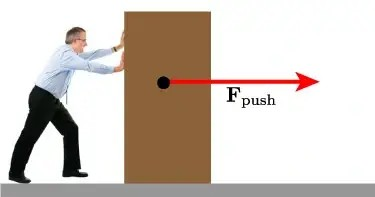# Why ‘Work’ is a Scalar quantity? A short story

27/03/2022Credit image: public image

Article by: Abhinav Tanksale, on 27 March 2022, at 08:32 am PDT

You might have learned during high school that Work = Force x Displacement

Now here's an interesting question. If both 'force' and 'displacement' are vector quantities then why is their product (work) a Scalar quantity? To answer this, we will use some fundamentals, a few examples and an interesting story! Ready?

Let's first begin by understanding the basic difference between a Vector and a Scalar.

Scalar quantities describe physical quantities that can be completely specified by a magnitude, or numerical value, as opposed to a vector quantity, which has both a magnitude and a direction. For example, temperature is a scalar quantity because a temperature of 100 degrees is the same as a temperature of -100 degrees. The temperature has a magnitude of 100 but no direction.

Similarly, Work is a quantity that is equal to the magnitude of a vector (force) that you are applying times the distance in which you are applying the force.

Confused? If you are using a vector to determine the direction, there are two different ways that you could be working. If you are moving an object with a force, the work being done is the force times the distance that the object was moved.It is easier to remember that positive work is when you lift an object and negative work is when you push an object.

If you are not moving an object, then you are doing zero work, no matter how much force you are exerting. In short, a scalar quantity is a quantity that does not have any direction associated with it. One more example would be, a number or mass. On the other hand, vector quantity has both magnitude and direction (like Force, Velocity, and Acceleration).

But, is there any mathematical proof/statement to validate this phenomenon? Yes, there is! and to understand how it works, here's an interesting story.

Long ago, in the realm of Science, there lived a civilization called Physics.

The citizens (quantities) of physics always fought with each other over the possession of a throne- The Throne of VECTOR.

Unlike the throne of SCALAR, which belonged to citizens who had Strength (magnitude) in them, the throne of VECTOR belonged to citizens who possessed Strength as well as Vision (direction) to guide the civilization.

An ambitious citizen called WORK desired to claim the VECTOR Throne. He was the son of two citizens of the Vector caste - Force & Displacement. But, he was banned from acquiring it & instead was given the Throne of the scalar kingdom.

Work became angry & went to God (Mathematics) for help.

God said, "You are a dot product of force & displacement & the Dot product of two vectors is always a Scalar."

That's why Work remains a Scalar quantity today.